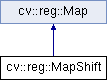OpenCV  3.0.0 Open Source Computer Vision
cv::reg::MapShift Class Reference

#include "mapshift.hpp"

Inheritance diagram for cv::reg::MapShift:## Public Member Functions

MapShift (void)

MapShift (const cv::Vec< double, 2 > &shift)

~MapShift (void)

void compose (const Map &map)

const cv::Vec< double, 2 > & getShift () const

cv::Ptr< MapinverseMap (void) const

void inverseWarp (const cv::Mat &img1, cv::Mat &img2) const

void scale (double factor)Public Member Functions inherited from cv::reg::Map
virtual ~Map (void)

virtual void warp (const cv::Mat &img1, cv::Mat &img2) const

## Detailed Description

Defines an transformation that consists on a simple displacement

## Constructor & Destructor Documentation

 cv::reg::MapShift::MapShift ( void )

Default constructor builds an identity map

 cv::reg::MapShift::MapShift ( const cv::Vec< double, 2 > & shift )

Constructor providing explicit values

Parameters
 [in] shift Displacement
 cv::reg::MapShift::~MapShift ( void )

Destructor

## Member Function Documentation

 void cv::reg::MapShift::compose ( const Map & map )
virtual

Changes the map composing the current transformation with the one provided in the call. The order is first the current transformation, then the input argument.

Parameters
 [in] map Transformation to compose with.

Implements cv::reg::Map.

 const cv::Vec& cv::reg::MapShift::getShift ( ) const
inline

Return displacement

Returns
Displacement
 cv::Ptr cv::reg::MapShift::inverseMap ( void ) const
virtual

Calculates the inverse map

Returns
Inverse map

Implements cv::reg::Map.

 void cv::reg::MapShift::inverseWarp ( const cv::Mat & img1, cv::Mat & img2 ) const
virtual

Warps image to a new coordinate frame. The calculation is img2(x)=img1(T(x)), so in fact this is the inverse warping as we are taking the value of img1 with the forward transformation of the points.

Parameters
 [in] img1 Original image [out] img2 Warped image

Implements cv::reg::Map.

 void cv::reg::MapShift::scale ( double factor )
virtual

Scales the map by a given factor as if the coordinates system is expanded/compressed by that factor.

Parameters
 [in] factor Expansion if bigger than one, compression if smaller than one

Implements cv::reg::Map.

The documentation for this class was generated from the following file:
• /builds/master-contrib_docs-mac/opencv_contrib/modules/reg/include/opencv2/reg/mapshift.hpp